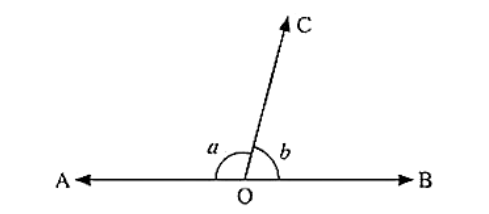# In Fig: a is greater than b by one third of a right angle. Find the value of a and b?

Question:

In Fig: a is greater than b by one third of a right angle. Find the value of a and b?Solution:

Since a and b are linear

a + b = 180

a = 180 – b  ... (1)

From given data, a is greater than b by one third of a right angle

a = b + 90/3

a = b + 30

a – b = 30 ... (2)

Equating (1) and (2)

180 – b = b + 30

180 – 30 = 2b

b = 150 / 2

b = 75

From (1)

a = 180 – b

a = 180 – 75

a = 105

Hence the values of a and b are 105° and 75° respectively.